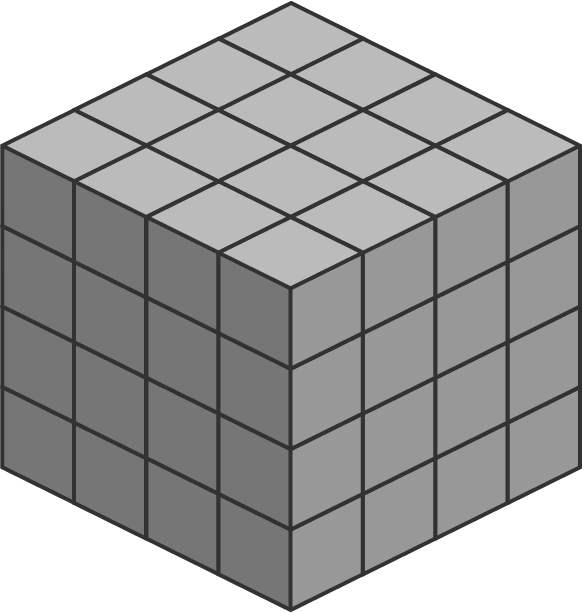# Tic-Tac-Toe to the Nth Dimension

Probability Level 3

In a normal $3 \times 3$ tic-tac-toe board, there are 8 winning lines.How many winning lines are there in a $4\times 4\times 4$ tic-tac-toe board? (In this case, a winning line consists of four boxes in a row, either on the surface or inside the cube.)Bonus: How many winning lines are there in a $n^d$ tic-tac-toe hypercube, where $n$ is the number of cells per side and $d$ is the number of dimensions?

×